A step to combine the technology and spiritualism.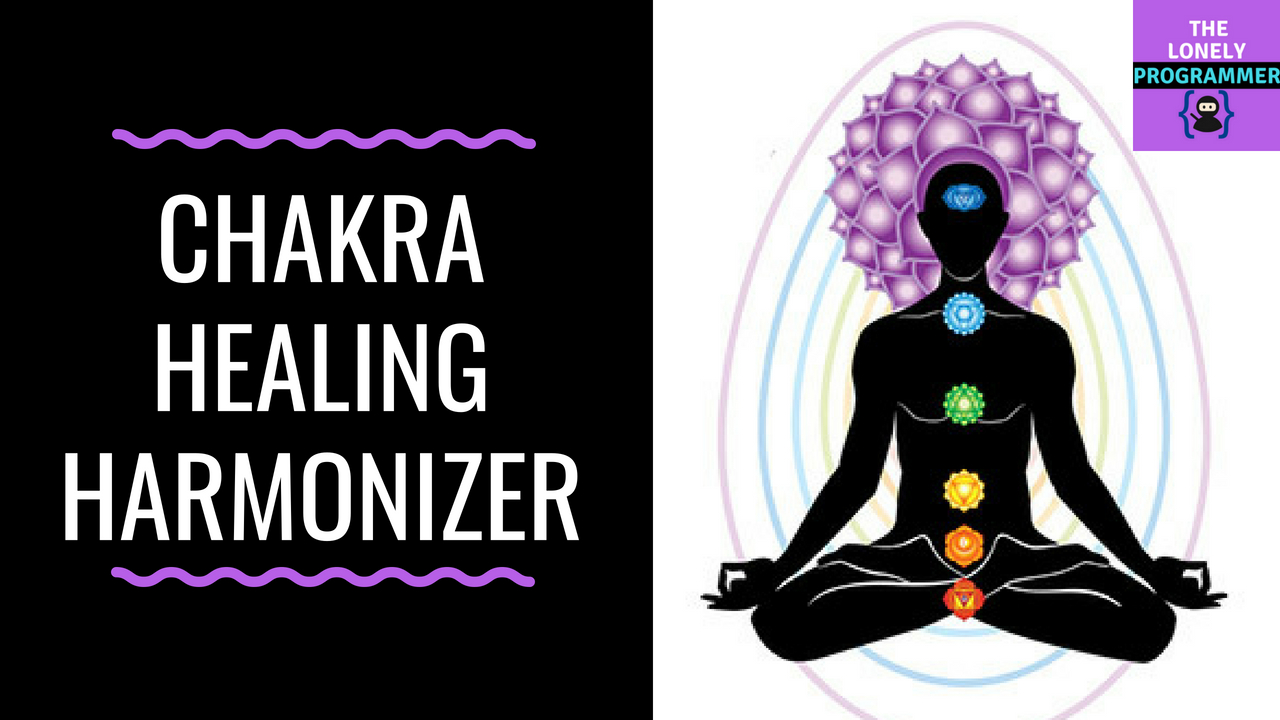A step to combine the technology and spiritualism.

This project demonstrates the use of electronics and embedded system with Chakra Meditation.

This is my step to put forth to help a yoga institution and to create an awareness about Chakra healing.

## Step 1: Parts and Material

Arduino Uno x 1

Relay Module x 7

Arduino MP3 Shield x 1

Speaker: 3W, 4 ohms x 2

RGB LED panel x 7

## Step 2: Hardware

I had cut the Plywood to the required dimension and Glass to cover each RGB LED Panel.

The 12V adapter is installed for powering the device and a separate adapter is used to light the LED Panel. The Relay Connections are used to switch the LED Color Panels. Make sure that the supply is isolated.

Drill the plywood to mount the PCB.

TX -> RX

RX -> TX

VCC -> 3.3v

GND -> GND

IN1 -> A1

IN2 -> A2

IN3 -> A3

IN4 -> A4

IN5 -> A5

IN6 -> A6

IN7 -> A7

VCC -> VCC

GND -> GND

TX -> D10

RX -> D11

VCC -> 3.3v

GND -> GND

## Step 4: Code

You can find the code below

## Step 5: Connections

You can find the connections below

## Step 6: Application

For now we will use the ready-made application. In the following days I will publish the application once after the certificates are processed.

Working of the Project: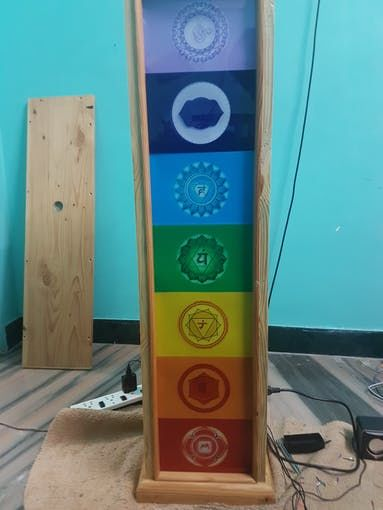The Harmonizer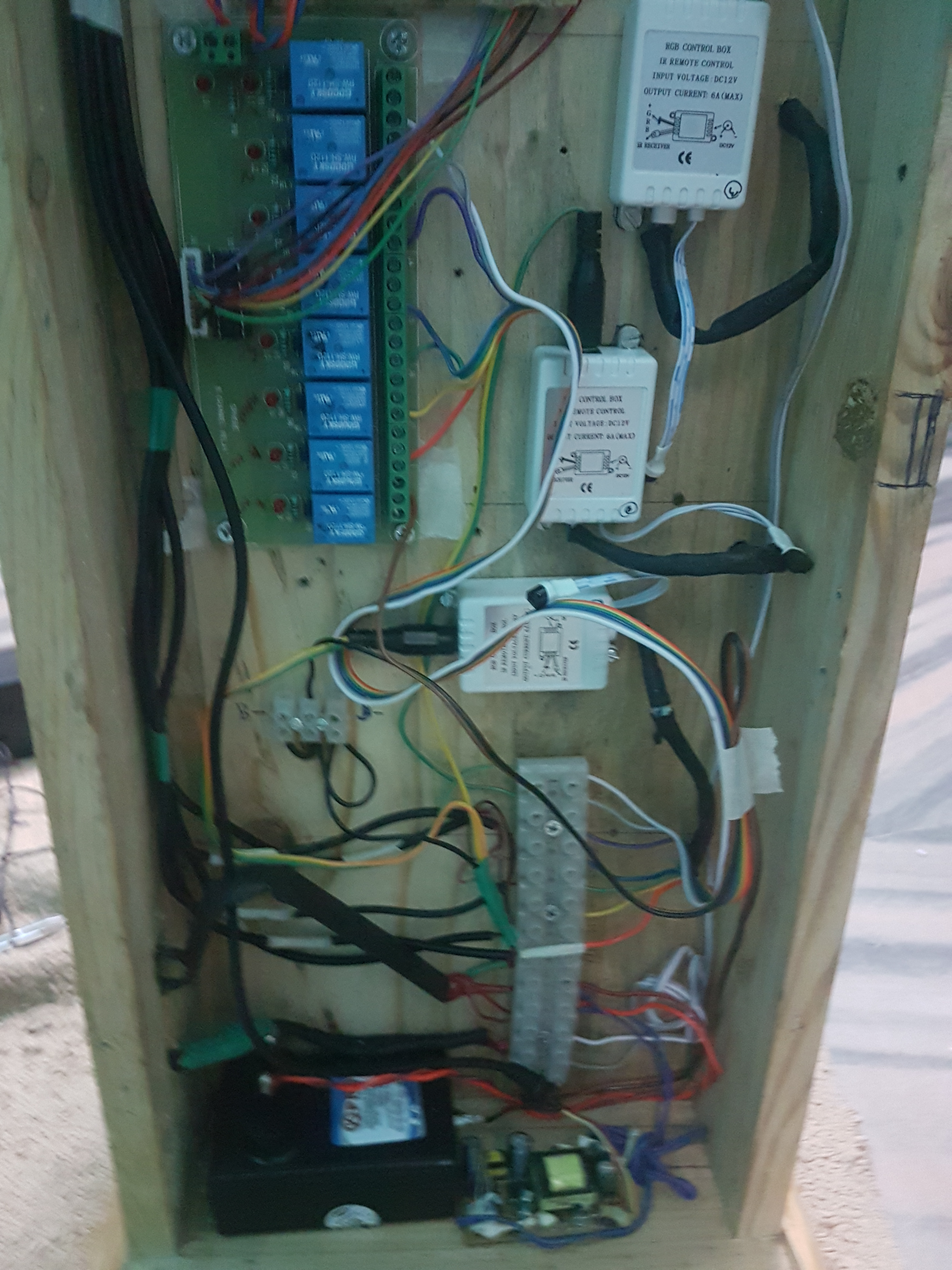Wiring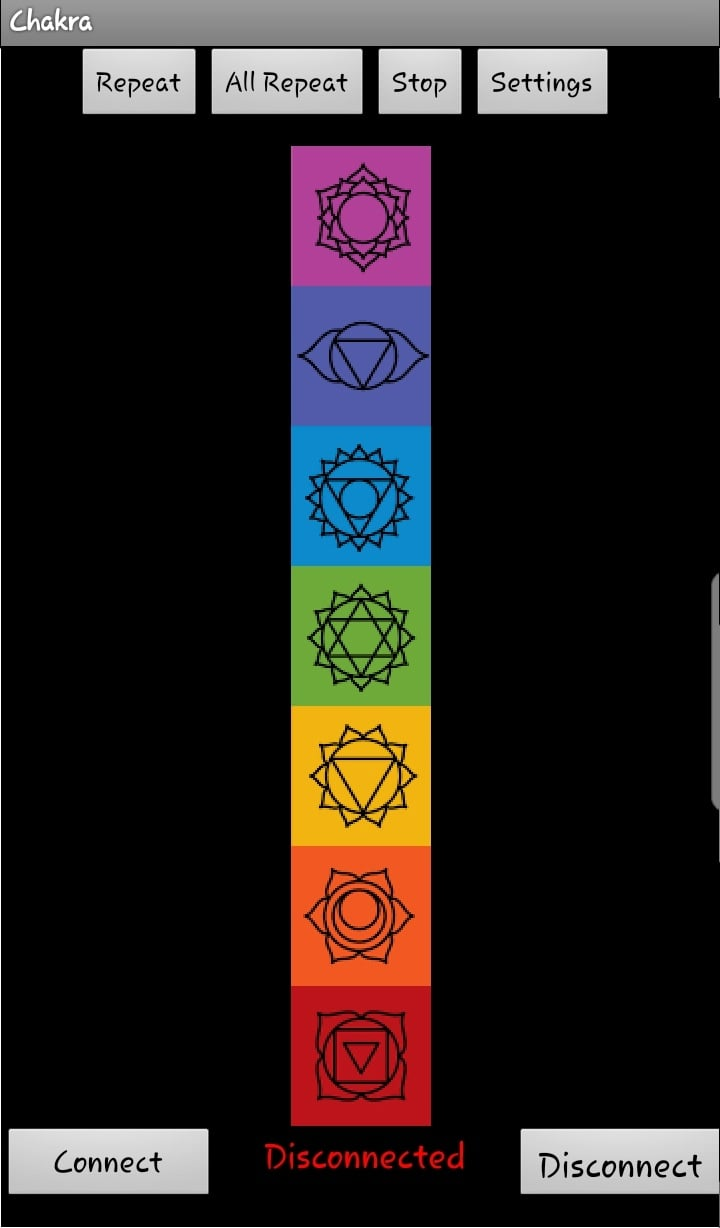App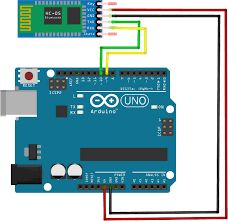Bt connection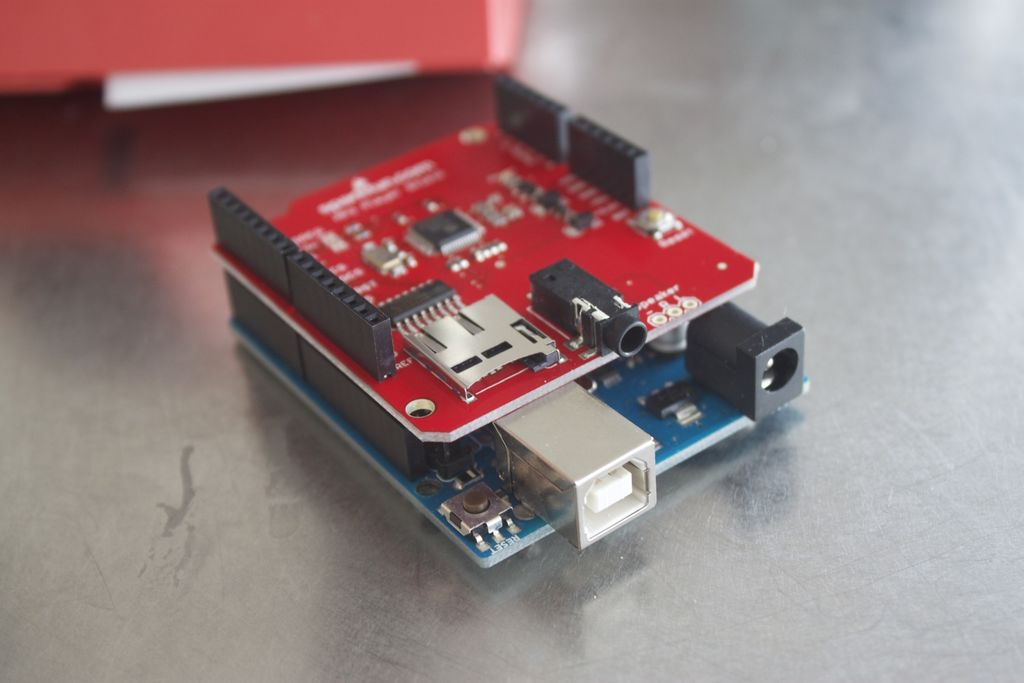Mp3 module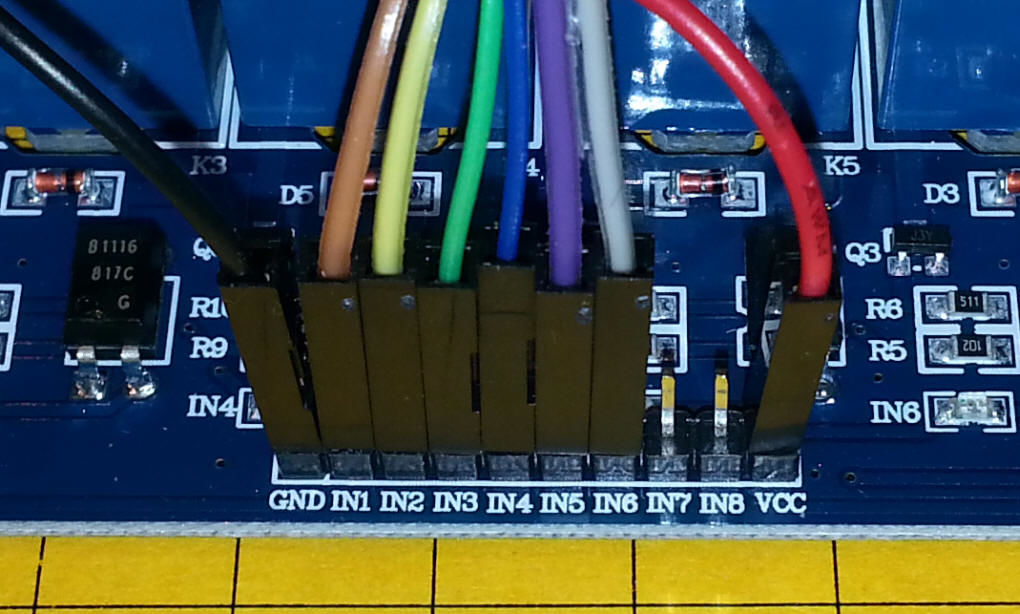Relay board

```        ```//Chakra Healing Harmonizer using Arduino

/*

Visit the Channel for more interesting projects

*/
#include "SoftwareSerial.h"
#include <DFPlayer_Mini_Mp3.h>

SoftwareSerial mySerial(11, 10);
# define Start_Byte 0x7E
# define Version_Byte 0xFF
# define Command_Length 0x06
# define End_Byte 0xEF
# define Acknowledge 0x00  info]

# define ACTIVATED LOW
boolean isPlaying = false;

int but = 12;
int r1= A1, r2 = A2, r3 = A3, r4 = A4, r5 = A5, r6 = A6, r7 = A7;

void setup () {
pinMode(but, INPUT_PULLUP);
digitalWrite(but,HIGH);

pinMode(r1, OUTPUT);
digitalWrite(r1,LOW);
pinMode(r2, OUTPUT);
digitalWrite(r2,LOW);
pinMode(r3, OUTPUT);
digitalWrite(r3,LOW);
pinMode(r4, OUTPUT);
digitalWrite(r4,LOW);
pinMode(r5, OUTPUT);
digitalWrite(r5,LOW);
pinMode(r6, OUTPUT);
digitalWrite(r6,LOW);
pinMode(r7, OUTPUT);
digitalWrite(r7,LOW);

Serial.begin(9600);
mySerial.begin (9600);
mp3_set_serial (mySerial);
mp3_set_volume (25);
}

void loop () {
check_bt();
}

void check_bt()
{
while (Serial.available()){
delay(10);
if ((c == '~')|| (c == '+')) {break;}
voice += c;
}
if (voice.length() > 0) {
Serial.println(voice);

if(voice == "playc1")
{
voice=""; p1 = 1;relay_1();
mp3_play (1);
wait(hr1,minute1,0);
mp3_stop ();
}
else p1 = 0;

if(voice == "playc2")
{
voice=""; p2 = 1;relay_2();
mp3_play (2);
wait(hr2,minute2,0);
mp3_stop ();
}
else p2 = 0;

if(voice == "playc3")
{
voice=""; p3 = 1;relay_3();
mp3_play (3);
wait(hr3,minute3,0);
mp3_stop ();
}
else p3 = 0;

if(voice == "playc4")
{
voice=""; p4 = 1;relay_4();
mp3_play (4);
wait(hr4,minute4,0);
mp3_stop ();
}
else p4 = 0;

if(voice == "playc5")
{
voice=""; p5 = 1;relay_5();
mp3_play (5);
wait(hr5,minute5,0);
mp3_stop ();
}
else p5 = 0;

if(voice == "playc6")
{
voice=""; p6 = 1;relay_6();
mp3_play (6);
wait(hr6,minute6,0);
mp3_stop ();
}
else p6 = 0;

if(voice == "playc7")
{
voice=""; p7 = 1;relay_7();
mp3_play (7);
wait(hr7,minute7,0);
mp3_stop ();
}
else p7 = 0;

//////////////////////////////////////////////////////////////////////

if(voice == "playal")
{
voice=""; p_cur = 1;
if(p1 == 1)
{
p1 = 0; relay_1();
mp3_play (1);
wait(2,0,0);
mp3_stop ();
}
else if(p2 == 1)
{
p2 = 0; relay_2();
mp3_play (2);
wait(2,0,0);
mp3_stop ();
}
else if(p3 == 1)
{
p3 = 0; relay_3();
mp3_play (3);
wait(2,0,0);
mp3_stop ();
}
else if(p4 == 1)
{
p4 = 0; relay_4();
mp3_play (4);
wait(2,0,0);
mp3_stop ();
}
else if(p5 == 1)
{
p5 = 0; relay_5();
mp3_play (5);
wait(2,0,0);
mp3_stop ();
}
else if(p6 == 1)
{
p6 = 0; relay_6();
mp3_play (6);
wait(2,0,0);
mp3_stop ();
}
else if(p7 == 1)
{
p7 = 0; relay_7();
mp3_play (7);
wait(2,0,0);
mp3_stop ();
}

}
else p_cur = 0;

/////////////////////////////////////////////////////////////////////////////
if(voice == "playrc")
{
voice=""; p_order = 1;
p1 = 1; relay_1();
mp3_play (1);
wait(hr1,minute1,0);
p1 = 0;
mp3_stop ();
wait(0,1,0);

p2 = 1; relay_2();
mp3_play (2);
wait(hr2,minute2,0);
p2 = 0;
mp3_stop ();
wait(0,1,0);

p3 = 1; relay_3();
mp3_play (3);
wait(hr3,minute3,0);
p3 = 0;
mp3_stop ();
wait(0,1,0);

p4 = 1; relay_4();
mp3_play (4);
wait(hr4,minute4,0);
p4 = 0;
mp3_stop ();
wait(0,1,0);

p5 = 1; relay_5();
mp3_play (5);
wait(hr5,minute5,0);
p5 = 0;
mp3_stop ();
wait(0,1,0);

p6 = 1; relay_6();
mp3_play (6);
wait(hr6,minute6,0);
p6 = 0;
mp3_stop ();
wait(0,1,0);

p7 = 1; relay_7();
mp3_play (7);
wait(hr7,minute7,0);
p7 = 0;
mp3_stop ();
}
else p_order = 0;

if(voice == "playst") {mp3_stop (); relay_stop();
p1 = 0; p2 = 0; p3 = 0; p4 = 0; p5 = 0; p6 = 0; p7 = 0;
p_cur = 0; p_order = 0;}
voice="";}
}

void wait(int h,int m,int s)
{
int t = (h*60*60)+(m*60)+s;
for(int i=1;i<t;i++) //1sec 1000ms
{
for(int j=0;j<40;j++)
{
delay(25);
check_bt();
}
}
}

void relay_1()
{
digitalWrite(r1,HIGH);
digitalWrite(r2,LOW);
digitalWrite(r3,LOW);
digitalWrite(r4,LOW);
digitalWrite(r5,LOW);
digitalWrite(r6,LOW);
digitalWrite(r7,LOW);
}

void relay_2()
{
digitalWrite(r1,LOW);
digitalWrite(r2,HIGH);
digitalWrite(r3,LOW);
digitalWrite(r4,LOW);
digitalWrite(r5,LOW);
digitalWrite(r6,LOW);
digitalWrite(r7,LOW);
}

void relay_3()
{
digitalWrite(r1,LOW);
digitalWrite(r2,LOW);
digitalWrite(r3,HIGH);
digitalWrite(r4,LOW);
digitalWrite(r5,LOW);
digitalWrite(r6,LOW);
digitalWrite(r7,LOW);
}
void relay_4()
{
digitalWrite(r1,LOW);
digitalWrite(r2,LOW);
digitalWrite(r3,LOW);
digitalWrite(r4,HIGH);
digitalWrite(r5,LOW);
digitalWrite(r6,LOW);
digitalWrite(r7,LOW);
}

void relay_5()
{
digitalWrite(r1,LOW);
digitalWrite(r2,LOW);
digitalWrite(r3,LOW);
digitalWrite(r4,LOW);
digitalWrite(r5,HIGH);
digitalWrite(r6,LOW);
digitalWrite(r7,LOW);
}

void  relay_6()
{
digitalWrite(r1,LOW);
digitalWrite(r2,LOW);
digitalWrite(r3,LOW);
digitalWrite(r4,LOW);
digitalWrite(r5,LOW);
digitalWrite(r6,HIGH);
digitalWrite(r7,LOW);
}

void relay_7()
{
digitalWrite(r1,LOW);
digitalWrite(r2,LOW);
digitalWrite(r3,LOW);
digitalWrite(r4,LOW);
digitalWrite(r5,LOW);
digitalWrite(r6,LOW);
digitalWrite(r7,HIGH);
}

void relay_stop()
{
digitalWrite(r1,LOW);
digitalWrite(r2,LOW);
digitalWrite(r3,LOW);
digitalWrite(r4,LOW);
digitalWrite(r5,LOW);
digitalWrite(r6,LOW);
digitalWrite(r7,LOW);
}
//*************************************************************************************************************************************
void playFirst()
{
execute_CMD(0x3F, 0, 0);
delay(100);
setVolume(25);
delay(100);
execute_CMD(0x11,0,1);
delay(100);
}

void initiate(){
execute_CMD(0x3F, 0, 0);
delay(100);
setVolume(25);
delay(100);}

void pause()
{
execute_CMD(0x0E,0,0);
delay(100);
}

void play()
{
execute_CMD(0x0D,0,1);
delay(100);
}

void playNext()
{
execute_CMD(0x01,0,1);
delay(100);
}

void playPrevious()
{
execute_CMD(0x02,0,1);
delay(100);
}

void setVolume(int volume)
{
execute_CMD(0x06, 0, volume);
delay(2000);
}

void play1()
{
relay_1();
execute_CMD(0x0D,0,1);
delay(100);
isPlaying = true;      wait(hr1,minute1,0);
pause();
}

void play2()
{
p2 = 1;
relay_2();
execute_CMD(0x0D,0,2);
delay(100);
isPlaying = true;      wait(hr2,minute2,0);
pause();
p2 = 0;
}

void play3()
{
p3 = 1;
relay_3();
execute_CMD(0x0D,0,3);
delay(100);
isPlaying = true;      wait(hr3,minute3,0);
pause();
p3 = 0;
}

void play4()
{
p4 = 1;
relay_4();
execute_CMD(0x0D,0,4);
delay(100);
isPlaying = true;      wait(hr4,minute4,0);
pause();
p4 = 0;
}

void play5()
{
p5 = 1;
relay_5();
execute_CMD(0x0D,0,5);
delay(100);
isPlaying = true;      wait(hr5,minute5,0);
pause();
p5 = 0;
}

void play6()
{
p6 = 1;
relay_6();
execute_CMD(0x0D,0,6);
delay(100);
isPlaying = true;      wait(hr6,minute6,0);
pause();
p6 = 0;
}

void play7()
{
p7 = 1;
relay_7();
execute_CMD(0x0D,0,7);
delay(100);
isPlaying = true;      wait(hr7,minute7,0);
pause();
p7 = 0;
}

void execute_CMD(byte CMD, byte Par1, byte Par2)
{
word checksum = -(Version_Byte + Command_Length + CMD + Acknowledge + Par1 + Par2);
byte Command_line = { Start_Byte, Version_Byte, Command_Length, CMD, Acknowledge,
Par1, Par2, highByte(checksum), lowByte(checksum), End_Byte};
for (byte k=0; k<10; k++)
{
mySerial.write( Command_line[k]);
}
}```
```

## More projects

Chakra Healing Harmonizer using Arduino: https://www.youtube.com/watch?v=fa5KX7VIZOs

Arduino Tutorial: J.A.R.V.I.S v1 | How to make a Home Automation: https://www.youtube.com/watch?v=QZbFyd1MMxo

Tamil Library for 16x2 LCD - தமிழ் எழுத்துக்கள்: https://www.youtube.com/watch?v=ph81PUIQe7w

Give a thumbs up if it really helped you and do follow my channel for interesting projects :)